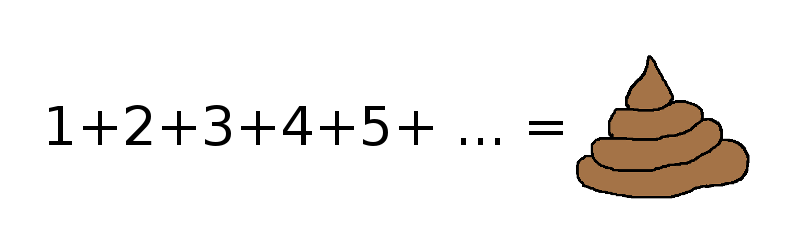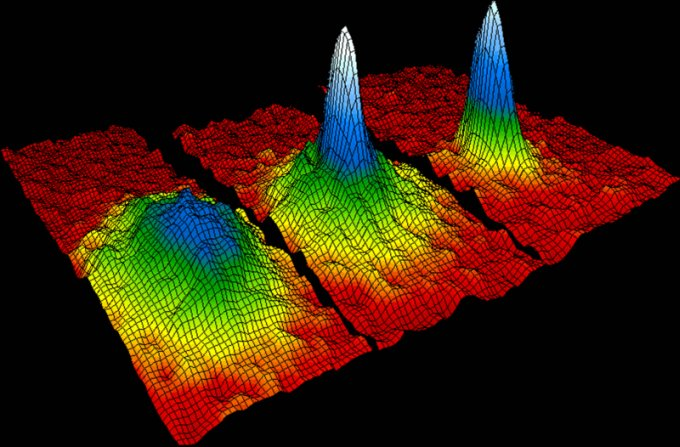1 + 2 + 3 + 4 + 5 + ... = -1/12? Bollocks!

Updated: February 21, 2014

Recently, I've come across a Youtube video proving that the sum of all natural numbers is -1/12. Now, your first reaction to this kind of claim is a mild WTF, which is exactly the intention. But if you're an educated person, you will spend a few moments watching, figuring out the fallacy in the claim, and move on. If you're an impressionable little git, you will spam everyone and their sister with the link to zomg! amazing! video.

Since I was forced to endure more than one reference to this Youtube clip, I decided to write about it and shed my own pure rainbow sunshine onto the world. And so, this article joins a very long series of other bollocks articles, each and every one shattering a popular myth, misconception or other silly claim. Here we are.## Why this is simply not true

The actually proof looks convincing, but there are some big problems with it. First, it starts with a kind of a lemma, which is supposed to guide us toward our solution in a wicked, populistic sort of a way. Namely, the infinite sum of the Grandi's Series, 1 - 1 + 1 - 1 + ... which amounts to a staggering 1/2. After that, the proof continues with a Cesaro summation of a bunch of these Grandi's series, which ain't your intuitive algebraic sum you expect, with one of them being shifted by a member toward infinity. No biggie you say, since we have an infinite number of members. And so forth. Eventually, you get the nice result that everyone wants you to have. Or at least the super-turbo-scientists among them.

Let's begin with the first proof, that of a sum of a series of ones with the alternating sign. The proof says the sum will either be 0 or 1, but since we not know how many members are, we assume average, which is 1/2. Okay, my problem starts here.

How can a sum of natural numbers be a non-natural number? If so, we should include all fractional expressions available, and then it's no longer just integers. Second, does not this kind of expression violate the actual properties of the mathematical space? I'm not enough of a mathematician to really check this, but seems to me that integer plus integer ought to be integer. Reading a bit about integers on Wikipedia, I found this:

The integers (with addition as operation) form the smallest group containing the additive monoid of the natural numbers. Like the natural numbers, the integers form a countably infinite set. In algebraic number theory, these commonly understood integers, embedded in the field of rational numbers, are referred to as rational integers to distinguish them from the more broadly defined algebraic integers. The integers (with addition and multiplication addition) form a unital ring which is the most basic one, in the following sense: for any unital ring, there is a unique ring homomorphism from the integers into this ring. This universal property characterizes the integers.

The only algebraic integers which are found in the set of rational numbers are the integers. In other words, the intersection of Q and A is exactly Z. The rational number a/b is not an algebraic integer unless b divides a. Note that the leading coefficient of the polynomial bx ? a is the integer b. As another special case, the square root ?n of a non-negative integer n is an algebraic integer, and so is irrational unless n is a perfect square.

So perhaps it can't be. And we're dealing with all numbers. In which case, we should also sum 1.01 and 1.05 and 1.884 and all the rest of them, and then, the actual proof becomes something else entirely. Furthermore, the sum might approach 1/2 in some theoretical vacuum, but we do not know how, and the original question does not define the rules of the game. A continuous function? A quantized field? Maybe. The rate of change? Eigenvalues? Yup, I go back to my claim. Finally, let us not forget the actual limitations of the physical world we live in. Silly me.

But let us say this CAN happen, even thought this is a divergent series, so the infinite sum does not actually yield anything meaningful. Never mind. Let's try the same trick with something else. Electron spin values. As you know, in its minimal energy state, the electron spin can be either 1/2 or -1/2. But you can't really know until you measure it. Therefore, if we sum all electrons in the world, on average, their spin is zero. Which means they no longer have half-integer spin values, they are no longer fermions, they do not obey the Pauli exclusion principle, and they are bosons really. Only you can't have electrons assume the state of Bose-Einstein Condensate.Now, you may say, on a macroscopic scale, this is exactly what happens. Except no. Perhaps some of the quantum effects can't be observed by naked eye and such, but they are still valid. And on average, electrons obey the Pauli exclusion principle, and this is what governs the way the nature is built, and elements in the periodic table tend to agree. Ergo, the averaging of spin values does not hold water. Next.

Shifting a series by one member. Not bad, but my question is, if you represent these series as matrices, or vectors if you will, what takes the place of a displaced member? Do you have a vector index that is undefined right now? Are you not changing the rules of the space again? So we have an NA element, and we sum it with what exactly? We offset the vector. We transformed the space. Sure, we can use the phrase infinity to put a kind of a long tail to everything, but this doesn't change anything. You can't sum an integer with an undefined quantity it and still call it integer.

When you take these two problems and ignore them, sure the series will behave they want it to behave. Otherwise, you can shift backwards. Or skip two members. Why not? Now, I might be a math idiot, but reading the following paragraphs from the Grandi's series article made me thinking:

In modern mathematics, the sum of an infinite series is defined to be the limit of the sequence of its partial sums, if it exists. The sequence of partial sums of Grandi's series is 1, 0, 1, 0, ..., which clearly does not approach any number (although it does have two accumulation points at 0 and 1). Therefore, Grandi's series is divergent.

It can be shown that it is not valid to perform many seemingly innocuous operations on a series, such as reordering individual terms, unless the series is absolutely convergent. Otherwise these operations can alter the result of summation.

So maybe not. And someone did it for the lulz to stir up some fire in the community.

## Conclusion

Here we are. I am sure a nice, smart mathematician might read this and then Mongol-molest this article to pieces, but from what little I know of math and physics, and I have no problem with the non-intuitive nature of the quantum mechanics, this proof does not seem legit to me. The pure math is correct, but the reality is wrong. It simply assumes a whole bunch of things that aren't there, it ignores the rules of algebra and some more in the process.

The biggest problem is the shifting of the series, which goes against what you may do with divergent series. And finally, it cannot be applied to physics, as I've shown you with my electron spin thingie. Very popular in the blogosphere and all that, but overall, we must classify this proof as bollocks. And we're done.

Cheers.